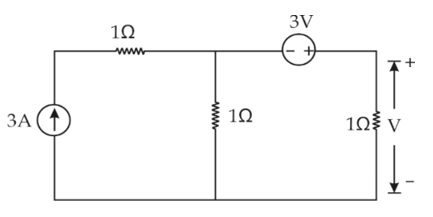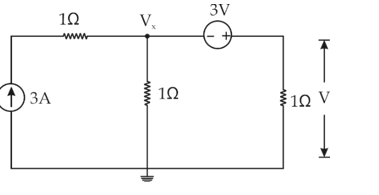Engineering Jobs   »   DFCCIL ELECTRICAL QUIZ

# DFCCIL’21 EE: Daily Practices Quiz 05-Aug-2021

Each question carries 1 mark.
Negative marking: 1/4 mark
Total Questions: 06
Time: 08 min.

Q1. Consider the following statements with regard to the synchronous machine.
1. Star-delta starters are used to start synchronous motors.
2. Damper windings are used to prevent hunting.
The correct statement(s) is/are:
(a) 1 only
(b) 2 only
(c) Both 1 and 2
(d) Neither 1 nor 2

Q2. In a three-phase induction motor if the rotor input or air gap power is 20,000 W and slip is 3 %. The rotor copper loss per phase is:
(a) 600 W
(b) 100 W
(c) 200 W
(d) 300 W

Q3. A 50 KVA transformer has a core loss of 150 W and total loss of 400 W. Find the copper loss for maximum efficiency:
(a) 400 W
(b) 150 W
(c) 250 W
(d) 300 W

Q4. In a d.c. machine, the current rating and voltage rating of wave winding is
(a) Low, high
(b) High, low
(c) High, high
(d) Low, low

Q5. A forward bias PN junction will act as a/an:
(a) Amplifier
(b) Open switch
(c) Closed Switch
(d) Attenuator

Q6. The value of V in the circuit shown in the given figure is(a) 1 V
(b) 2 V
(c) 3 V
(d) 4 V

SOLUTIONS
S1. Ans.(b)
Sol. Synchronous motors are not self-starting.
Starting methods:
Starting with the help of an External Prime Mover
Starting with the help of Damper Windings
Damper windings are used to prevent the rotor from losing synchronism in a synchronous machine and this phenomenon is called Hunting.

S2. Ans.(c)
Sol. Rotor Cu loss=sP_in=0.03×20,000=600 W
∴Rotor Cu loss per phase=600/3=200 W.

S3. Ans.(b)
Sol. For maximum efficiency: Copper loss=iron or core loss=150 W

S4. Ans.(a)
Sol. wave winding is used for HIGH VOLTAGE and low current rating applications.

S5. Ans.(c)
Sol. If the positive terminal of an external battery is connected to p-type and its negative terminal to n-type of PN junction then such a biasing called forward biasing.
An ideal diode acts as a short circuit or closed switch under forward bias condition and open circuit or open switch under reverse biased condition.

S6. Ans.(c)
Sol. Let the node voltage be V_x.Applying KCL at the given node, we have:
V_x/1+(V_x+3)/1=3
or V_x = 0 V
∴V_x+3=V
Or V=3 V

Sharing is caring!